StatLect

# Normal equations

In linear regression analysis, the normal equations are a system of equations whose solution is the Ordinary Least Squares (OLS) estimator of the regression coefficients.

The normal equations are derived from the first-order condition of the Least Squares minimization problem.## Simple linear regression

Let us start from the simple linear regression model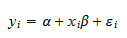where:

•is the dependent variable;

•is the constant (or intercept);

•is the regressor;

•is the regression coefficient (or slope);

•is the zero-mean error term.

There areobservations in the sample:.

### Normal equations in the simple regression model

The normal equations for the simple regression model are: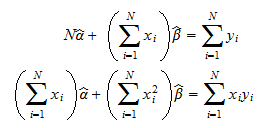whereand(the two unknowns) are the estimators ofand.

Proof

The OLS estimators ofand, denoted byand, are derived by minimizing the sum of squared residuals: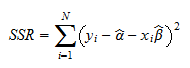We carry out the minimization by computing the first-order conditions for a minimum. In other words, we calculate the derivatives ofwith respect toand, and we set them equal to zero: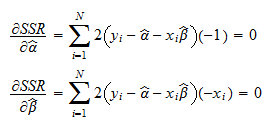We divide the two equations byand obtain the equivalent system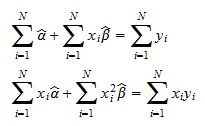Sincewe can write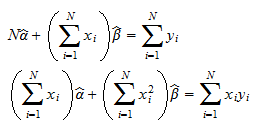which are the two normal equations displayed above.

Thus, in the case of a simple linear regression, the normal equations are a system of two equations in two unknowns (and).

If the system has a unique solution, then the two values ofandthat solve the system are the OLS estimators of the interceptand the sloperespectively.

## Multiple linear regression

In a multiple linear regression, in which there is more than one regressor, the regression equation can be written in matrix form:where:

•is thevector of dependent variables;

•is thematrix of regressors (the so-called design matrix);

•is thevector of regression coefficients;

•is thevector of error terms.

### Normal equations in the multiple regression model

The normal equations for the multiple regression model are expressed in matrix form aswhere the unknownis avector (the estimator of).

Proof

The OLS estimator of the vector, denoted by, is derived by minimizing the sum of squared residuals, which can be written in matrix form as follows: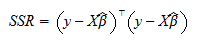In order to find a minimizer, we compute the first-order condition for a minimum. We calculate the gradient of(the vector of partial derivatives with respect to the entries of) and we set it equal to zero:We divide the equations byand obtainwhich is a system of normal equations expressed in matrix form.

Thus, in the case of the multiple regression model, the normal equations, expressed above in matrix form, are a system ofequations inunknowns (theentries of the coefficient vector).

If the system has a unique solution, the value ofthat solves the system is the OLS estimator of the vector.

## How to solve the normal equations

As stated above, the normal equations are just a system oflinear equations inunknowns.

Therefore, we can employ the standard methods for solving linear systems.

For example, if the equations are expressed in matrix form and the matrixis invertible, we can write the solution as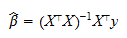## More details

More mathematical details about the normal equations and the OLS estimator can be found in these lectures:

## References

If you want to double check the formulae and the derivations shown above, you can check these references:

• Greene, W.H. (2003). Econometric analysis, Fifth Edition. Prentice Hall.

• Gujarati, D.N. (2004). Basic econometrics, Fourth Edition. McGraw-Hill.

Previous entry: Multinomial coefficient

Next entry: Null hypothesis